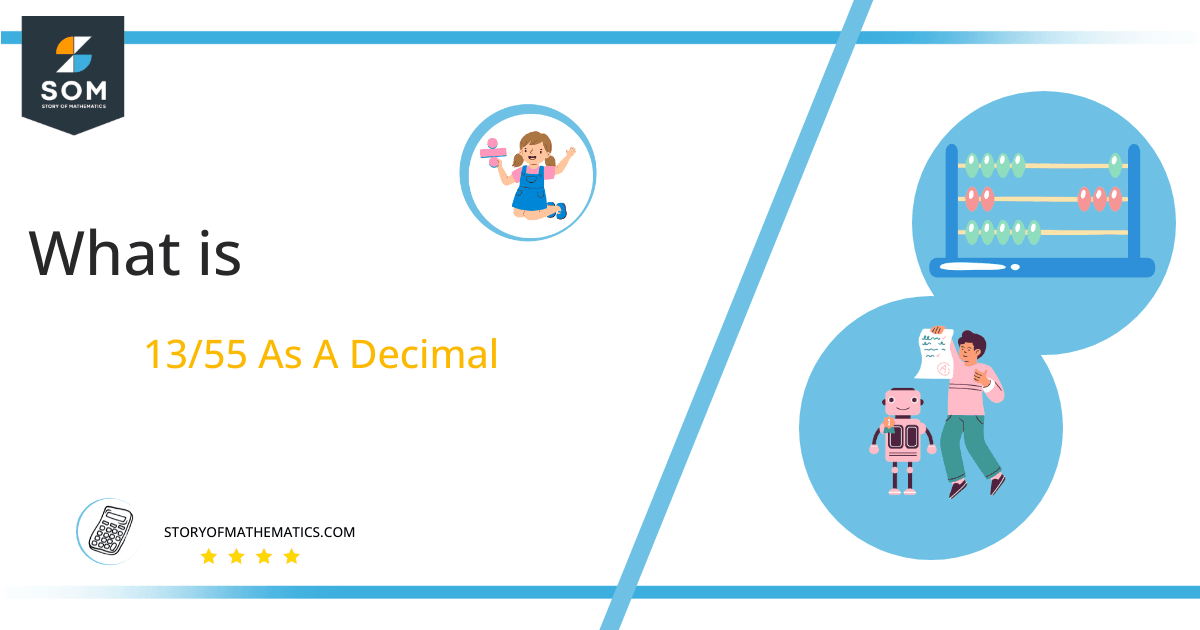# What Is 13/55 as a Decimal + Solution With Free Steps

The fraction 13/55 as a decimal is equal to 0.236.

When a thing is divided into various portions, the best way to represent these portions mathematically is through fractions. The fractions can be simple or complex.A simple fraction is one in which the numerator and denominator both are integers. In a complex fraction, either the numerator or denominator or both of them can be a fraction. In short, the complex fraction is the fraction that contains another fraction.

Here, we are more interested in the division types that result in a Decimal value, as this can be expressed as a Fraction. We see fractions as a way of showing two numbers having the operation of Division between them that result in a value that lies between two Integers.

Now, we introduce the method used to solve said fraction-to-decimal conversion, called Long Division, which we will discuss in detail moving forward. So, let’s go through the Solution of fraction 13/55.

## Solution

First, we convert the fraction components, i.e., the numerator and the denominator, and transform them into the division constituents, i.e., the Dividend and the Divisor, respectively.

This can be done as follows:

Dividend = 13

Divisor = 55

Now, we introduce the most important quantity in our division process: the Quotient. The value represents the Solution to our division and can be expressed as having the following relationship with the Division constituents:

Quotient = Dividend $\div$ Divisor = 13 $\div$ 55

This is when we go through the Long Division solution to our problem. Figure 1 contains the long division for the fraction 13/55.Figure 1

## 13/55 Long Division Method

We start solving a problem using the Long Division Method by first taking apart the division’s components and comparing them. As we have 13 and 55, we can see how 13 is Smaller than 55, and to solve this division, we require that 13 be Bigger than 55.

This is done by multiplying the dividend by 10 and checking whether it is bigger than the divisor or not. If so, we calculate the Multiple of the divisor closest to the dividend and subtract it from the Dividend. This produces the Remainder, which we then use as the dividend later.

Now, we begin solving for our dividend 13, which after getting multiplied by 10 becomes 130.

We take this 130 and divide it by 55; this can be done as follows:

130 $\div$ 55 $\approx$ 2

Where:

55 x 2 = 110

This will lead to the generation of a Remainder equal to 130 – 110 = 20. Now this means we have to repeat the process by Converting the 20 into 200 and solving for that:

200 $\div$ 55 $\approx$ 3

Where:

55 x 3 = 165

This, therefore, produces another Remainder equal to 200 – 165 = 35. Now we must solve this problem to the Third Decimal Place for accuracy, so we repeat the process with dividend 350.

350 $\div$ 55 $\approx$ 5

Where:

55 x 6 = 330

Finally, we have a Quotient generated after combining the three pieces of it as 0.236, with a Remainder equal to 20.Images/mathematical drawings are created with GeoGebra.